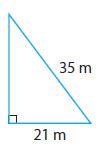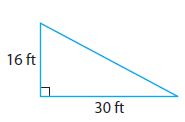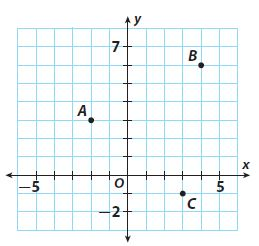Homework Explained - Math Practice 101Dear guest, you are not a registered member. As a guest, you only have read-only access to our books, tests and other practice materials.

As a registered member you can:

Registration is free and doesn't require any type of payment information. Click here to Register.
Go to page:
Chapter 12:The Pythagorean Theorem; Model Quiz

### 12.1 The Pythagorean Theorem

Find the length of the missing side.

• Question 1•  meters
• Question 2•  ft

### 12.2 Converse of the Pythagorean Theorem

Tell whether each triangle with the given side lengths is a right triangle.

• Question 7

Keelie has a triangular-shaped card. The lengths of its sides are 4.5 cm, 6 cm, and 7.5 cm. Is the card a right triangle?

### 12.3 Distance Between Two Points

Find the distance between the given points. Round to the nearest tenth.•  units
•  units
•  units

### ESSENTIAL QUESTION

• Question 11

How can you use the Pythagorean Theorem to solve real-world problems?

• Type below:

Yes, email page to my online tutor. (if you didn't add a tutor yet, you can add one here)